# MP Board Class 11th Chemistry Important Questions Chapter 11 p – Block Elements

## MP Board Class 11th Chemistry Important Questions Chapter 11 p – Block Elements

### p – Block Elements Important Questions

p – Block Elements Objective Type Questions

Question 1.
Choose the correct answer:

Question 1.
Boric acid is a polymer because:
(a) It is acidic in nature
(b) It contains H – bond
(c) Its basicity is one
(d) It has sheet like geometry
Answer:
(b) It contains H – bond

Question 2.
Which acid is not a protonic acid:
(a) H3BO3
(b) H3PO3
(c) H2SO3
(d) HCl3
Answer:
(a) H3BO3

Question 3.
Al2O3 can be converted to anhydrous AlCl3 by heating:
(a) Al2O3 with Cl2 gas
(b) Al2O3 with HCl gas
(c) Al2O3 with NaCl in solid state
(d) A mixture of Al2O3 and Carbon in dry Cl2 gas
Answer:
(d) A mixture of Al2O3 and Carbon in dry Cl2 gasQuestion 4.
Which one of the following is the correct statement:
(a) B2H6.2H3 is known as Inorganic benzene
(b) Boric acid is a protonic acid
(c) Beryllium exhibits co – ordination number of six
(d) Chlorides of both berylium and aluminium have bridged chloride structure in solid phase
Answer:
(d) Chlorides of both berylium and aluminium have bridged chloride structure in solid phase

Question 5.
Shape and hybridization of BF3 is:
(a) Linear, sp
(b) Planar, sp2
(c) Tetrahedral, sp3
(d) Pyrimidal, sp3
Answer:
(b) Planar, sp2

Question 6.
Reason for the formation of addition product between NH3 and BF3 is:
(a) Formation of H – bond between them
(b) Formation of ionic bond between them
(c) Formation of covalent bond between them
(d) Similar structure
Answer:
(c) Formation of covalent bond between them

Question 7.
Dry ice is:
(a) Solid ice without water
(b) Solid sulphur dioxide
(c) Solid carbon dioxide
(d) Solid benzene
Answer:
(c) Solid carbon dioxide

Question 8.
Which halide does not dissociate by water:
(a) CCl4
(b) SiCl4
(c) GeCl4
(d) SnCl2
Answer:
(a) CCl4Question 9.
Whose important constituent is silicon:
(a) Chlorophyll
(b) Haemoglobin
(c) Rocks
(d) Amalgam.
Answer:
(c) Rocks

Question 10.
Silicones are used:
(a) In the preparation of water proof cloth
(b) In the preparation of insulating material
(c) In preparing high elastic rubber
(d) All the above
Answer:
(d) All the above

Question 11.
Poisonous gas found in the smoke released from car:
(a) CH4
(b) C2H2
(C) CO
(d) CO2
Answer:
(C) CO

Question 2.
Fill in the blanks:

1. When formic acid is heated with cone. H2SO4, ………………………. is formed.
2. Bauxite ore containing ferric oxide as impurity is purified by ……………………… method.
3. Alumina containing both Fe2O3 and SiO2 as impurity is subjected to purification by ………………………… process.
4. To obtain cent percent pure aluminium, it is refined by ………………………….. process.
5. Melting point of pure alumina is very high (about 2050°C), to melt it at low temperature ………………………… and ………………………………. are added due to which melting point reduces to ……………………………..
6. When bauxite contains more of silica as impurity then the ore is purified by ………………………………….. process.
7. Process of catenation is maximum in ……………………………..
8. Fullerene is an aliotrope of ……………………………
9. Lamp black is an ………………………………. aliotrope of carbon.
10. Maximum covalency of carbon is ………………………… whereas of Si is …………………………
11. Graphite is a ……………………… of electricity where as silicon is a ……………………..
12. Silicon carbide is called …………………………….

Answer:

1. Carbon mono – oxide
2. Baeyer’s
3. Hall’s
4. Hoope’s
5. Cryolite, Fluorspar, 870°C
6. Serpeck
7. Carbon
8. Carbon
9. Amorphous
10. 4, 6
11. Conductor, semiconductor
12. CarborundumQuestion 3.
Answer in one word/sentence:

1. Which type of oxides are formed by elements of Group 13?
2. +1 oxidation state of T1 is more stable as compared to +3?
3. Aluminium reacts with base to form?
4. What are hydrides of boron known as?
5. Boron is m .inly found in which form?
6. What is “Two electron three centre bond” known as?
7. What will happen if excess of ammonia solution is added to copper sulphate solution?
8. What is formed when diborane react with ammonia?

Answer:

1. M2O3
2. Inert pair effect
3. Sodium metaaluminate
4. Borane
5. Borax (Na2B4O7.10H2O)
6. Diborane
7. Complex compound of cupric ammonium sulphite is formed
8. Borazine

Question 4.
Match the following:
[I]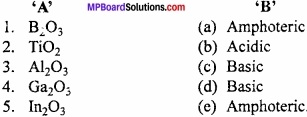Answer:

1. (b)
2. (c)
3. (e)
4. (a)
5. (d)

[II]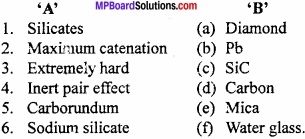Answer:

1. (e)
2. (d)
3. (a)
4. (b)
5. (c)
6. (f)

p – Block Elements Very Short Answer Type Questions

Question 1.
The +1 oxidation state of Tl is stable than +3 state?
Answer:
Inert pair effect.

Question 2.
What the hydride of boron is called?
Answer:
Borane.

Question 3.
Who is called “Two electron three centre bond”?
Answer:
Diborane.

Question 4.
What happens when ammonia solution is added in excess in CuSO4 solution?
Answer:
Complex compound of Cupric ammonium sulphate.Question 5.
What is the formula of Alum?
Answer:
K2SO4.Al2(SO4)3.24H2O.

Question 6.
What is the name of C60 carbon atom?
Answer:
Buckmister fullerenes.

Question 7.
Which carbide is harder than diamond?
Answer:
Boron carbide.

Question 8.
For which property of carbon, it have large number of compounds?
Answer:
Catenation.

Question 9.
What is the good conductor allotrope of carbon?
Answer:
Graphite.

Question 10.
What is water glass?
Answer:
Sodium silicate.

Question 11.
What is the hybridization of B in diborane?
Answer:
sp2.

Question 12.
What is carborundum?
Answer:
SiC (Silicon carbide).Question 13.
Which is electron deficient halide?
Answer:
BCl3.

Question 14.
What is the property of B2O3?
Answer:
Acidic nature.

Question 15.
What is dry ice?
Answer:
Solid CO2.

Question 16.
What is the formula of dimer of aluminium chloride?
Answer:
Al2Cl6.

Question 17.
What is used in welding as oxy acetylene flame?
Answer:
C2H2 (Acetylene).

Question 18.
What is Inorganic benzene?
Answer:
Borazene.

Question 19.
Which type of bond is present in diborane?
Answer:
2 electron 3 centre bond (Banana bond).Question 20.
Which type of hybridization in carbon atom found in diamond?
Answer:
sp2 hybridization.

Question 21.
Which is the most abundant metal found on earth surface?
Answer:
Aluminium.

p – Block Elements Short Answer Type Questions – I

Question 1.
How can you explain the higher stability of BCl3 as compared to TICI?
Answer:
Boron exhibits +3 oxidation state and can form stable BCl3. Thallium shows oxidation state of +1 as well as +3 but +1 oxidation state is more stable than +3 because of inert pair effect. Therefore, TlCl3 is not stable. It can form stable TlCl.

Question 2.
Consider the compounds, BCl3 and CCl4. How will they behave with water? Justify?
Answer:
The B atom in BCl3 has only six electrons in the valence shell and hence is an electron deficient molecule. It easily accepts a pair of electrons donated by water and hence BCl3 undergoes hydrolysis to form boric acid (H3BO3) and HCl.
BCl3 + 3H2O → H3BO3 + 3HCl
In contrast, C atom in CCl4 has 8 electrons in the valence shell. Therefore, it is an electron precise molecule. As a result, it neither accepts nor donates a pair of electrons from H2O molecule and hence CCl4 does not undergo hydrolysis in water.

Question 3.
Why caustic alkali like NaOH are not stored in aluminium vessel?
Answer:
Aluminium can easily dissolve in alkali and form sodium meta aluminate due to this reason caustic alkali like NaOH not stored in aluminium vessel.
2Al + 2NaOH + 2H2O → 2NaAlO2 + 3H2.Question 4.
What at normal temperature Al does not react with water?
Answer:
In the presence of air, a transparent protective oxide layer is formed on its surface, due to this at normal temperature it does not react with water.

Question 5.
Write the formula of Double salt or Alum?
Answer:
The general formula of double salt is R2SO4M2(SO4)3 where R = monovalent metal like Na, K, Rb, Cs or NH4+ ion and M is a trivalent metal like Fe+3, Al+3 or Cr+3.
Example: K2SO4.Al2(SO4)3.24H2O
(Potash Alum)

Question 6.
Write name of ores of aluminium? Write formulae also?
Answer:
Ores of aluminium are:

1. Oxides: Corundum, ruby, sapphire (Al2O3), emerald.
2. Hydrated oxides: Diaspore (Al2O3.H2O), bauxite (Al2O3.2H2O), gibbsite (Al2O3 – 3H2O).
3. Fluoride: Cryolite (Na3AlF6).
4. Sulphate: Alunite or alum stone [K2SO4.Al2(SO4)3.4Al(OH)3].
5. Silicates: Felspar (K2O.Al2O3.6SiO2).
6. Phosphate: Phiroza or turquoise [AlPO4. Al(OH)3]H2O.

Question 7.
Why aluminium is a strong reducing agent?
Answer:
Those elements which gives electron in the chemical reaction forms cations, and called reducing agent. The reducing power depends upon the electrode potential. (MPBoardSolutions.com) More the negative value of electrode potential more will be the reducing power. The electrode potential of A1 is – 1.67, so it behaves as a strong reducing agent.

Question 8.
Why gallium is a liquid at room temperature?
Answer:
Gallium changes into liquid when the room temperature is above 30°C. In solid state, its lattice energy is very less due to which the metallic bond can break easily at low temperature.Question 9.
Write the resonating structure of CO32- and HCO3?
Answer:
The resonance structure of CO32- is: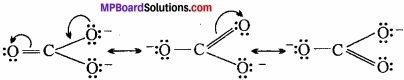Resonating structure of HCO3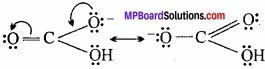Question 10.
Why the melting point and boiling point cf Boron is high?
Answer:
The crystal of Boron is formed by the covalent bond between the atoms. 2 atoms combines to form Icosahedron network which have 20 triangular faces and 12 comers. It makes boron very hard. Due to this it have high melting and boiling point.

Question 11.
What is Inorganic benzene? Why it is called Inorganic benzene?
Answer:
When diborane reacts with ammonia at 120°C forming an addition compound diammoniate of diborane B2H6.2NH3. When this diammoniate of diborane is heated at 200°C, a stable cyclic compound B3N 3H6 is formed.
Borazine B3N3H6 has cyclic structure similar to benzene and thus it is called inorganic benzene.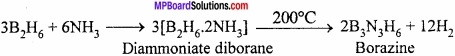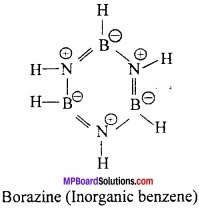Question 12.
What is corundum?
Answer:
Al is found in more than one crystalline forms. The most hardest crystal is known as corundum, which is used as abrasive.

Question 13.
Write the ores of Boron and write formula also?
Answer:
The ores of Boron are as follows:

1. Borax – Na2B4O7.10H2O
2. Kemite – Na2B4O7.2H2O
3. Colemanite – Ca2[B3O4.(0H)3]2.2H2O
4. Orthoboric acid – H3BO3.

Question 14.
Prove that Tl+3 is an oxidizing agent but Al+3 not?
Answer:
Due to inert pair effect, in boron family the stability of +1 oxidation state increases from top to bottom in a gap but the stability of +3 oxidation state decreases. (MPBoardSolutions.com) That is why in comparison to Tl+1 is more stable than Tl+3. Tl+3 + 2e $$\underrightarrow { \Delta }$$ Tl+1
It is clear that reduction of Tl+3 is occur. Therefore, Tl+3 is an oxidizing agent but the oxidation state of Al+3 is not possible.Question 15.
If B – Cl bond has a dipole moment, explain why BCl3 molecule has zero dipole moment?
Answer:
BCl3 has polar B – Cl bond, BCl3 is planar triangular molecule in which three B – Cl bonds are inclined at an angle of 120°. The resultant dipole moment of two B – Cl bonds is cancelled by the dipole moment of third B – Cl bond. The vector sum of the dipole moments of three B – Cl bond is zero.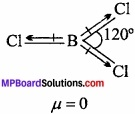Question 16.
Boric acid is not protic acid? Why?
Answer:
Protic acid is the one that gives protons in solutions. Boric acid not a protic acid because it does not ionize in water to give proton but it behaves as Lewis acid by accepting electron pair from hydroxide ion.
B(OH)3 + H2O ⇄ [B(OH)4] + H+

Question 17.
How borax is obtained from colemanite?
Answer:
Colemanite is boiled with concentrated sodium carbonate solution to get borax.
Ca2B6O11 + 2Na2CO3 → Na2B4O7 +2NaBO2 + 2CaCO3
On concentrating the product, crystals of borax is obtained. On passing CO2 in mother liquor, borax is obtained.
4NaBO2 + CO2 → Na2B4O7 + Na2CO3.

Question 18.
Why cryolite is used for the extraction of aluminium from alumina?
Answer:
The melting point of pure alumina is very high 2050°C, but in the presence of cryolite and fluorspar it melts at 870°C. In this way cryolite decreases the melting point of alumina. It also act as an electrolyte.Question 19.
Write the uses of carbon monoxide?
Answer:

1. It is main constituent of water gas (CO + H2) and producer gas (CO + N2).
2. Used for the preparation of some metal carbonyls.
3. It is used as reducing agents.

Question 20.
Why diamond is found rare in nature than graphite?
Answer:
The formation of diamond occurs at very high pressure and in liquid state of carbon converts into crystal. But in nature this state is very rare. That is why diamond is found rare in nature.

Question 21.
What is dry ice? Write its main uses?
Answer:
Solid carbon dioxide is called dry ice because its crystal looks like ice but they did not wet the paper and clothes. (MPBoardSolutions.com) At – 78.5°C it converts into solid without changing into liquid. It is used as coolant for preserving edibles and as anaesthetic agent in surgery.

Question 22.
What is carborundum? Write its main use?
Answer:
The structure of silicon carbide is hard like diamond. It is known as carborundum. It is used as abrasive for cutting tools.

Question 23.
Write the name of the compound used as coolant, anaesthetic and solvent, and write formula also?
Answer: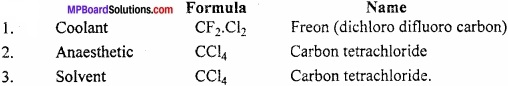Question 24.
Write the uses of graphite?
Answer:
The uses of graphite are as follows:

1. It is used in lead pencils in place of lead.
2. It is used as moderate in nuclear reactor.
3. Used as electrode in electrolytic and dry cells.

Question 25.
Write the different types of coal?
Answer:
The following types of coal are:

1. Pit: 60% carbon
2. Lignite: 70% carbon
3. Bituminous: 80% carbon
4. Anthracite: 90% carbon.

Question 26.
Write the uses of diamond?
Answer:
The uses of diamond are:

1. It is used for cutting or grinding of hard rocks.
2. It is used in high precision thermometer because it is a good conductor of heat.
3. For making windows of spaceships as it cuts off harmful radiations.Question 27.
Why CO2 is acidic? Explain with equation?
Answer:
The aqueous solution of CO2 is acidic:
CO2 + H2O → H2CO3
Carbonic acid
It turns blue litmus red and forms salt with base.
2NaOH + CO2 → Na2 CO3 + H2O
Ca(OH)2 + CO2 → CaCO3 + H2 O.

Question 28.
Why should not be sleep in a closed room keeping burning sigri?
Answer:
Sigri should not be bum in closed room, as in the smoke from sigri contains large amount of CO. This CO inhaled through respiration combines with the haemoglobin of blood and forms carboxyhaemoglobin which interrupt the blood flow and so the death occurs.

Question 29.
What are carbides?
Answer:
Carbides are those binary compounds of carbon which are formed by carbon with less electronegative atom.
They are of many types:

1. Ionic carbides
2. Metallic carbides
3. Interstitial carbides
4. Covalent carbides.

Question 30.
Write the use of silica gel?
Answer:
Silica gel is an amorphous solid which have 4% moisture. It is used as catalyst in petroleum industries. It is also used in chromatography.

Question 31.
What is Thixotropy?
Answer:
The viscosity of any liquid decreases by shaking it. This property is called Thixotropy. When SiCl4 is hydrolyzed at high temperature then a thixotropic silica is obtained.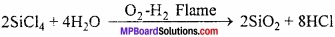Question 32.
What are interstitial carbides?
Answer:
When carbon atom get inserted in the interstitial places of crystal lattice of transition elements then the compound formed are called interstitial carbides. They are very hard and have high melting point.

Question 33.
What are methonides and acetanilides?
Answer:
Methonides:
The carbides after hydrolysis give methane are called methonides.
Al4C3 + 12H2O → 4Al(OH)3 + 3CH4
Acetanilides:
The carbides after hydrolysis gives acetylene are called acetanildes.
CaC2 + 2H2O → Ca(OH)2 + C2H2

Question 34.
Be and Ca are member of same group, but Ca forms CaC2 but Be forms Be2 C. Why?
Answer:
After the hydrolysis of CaC2 acetylene is formed, so its structure is CaC2 whereas after hydrolysis of Be2 C, methane is formed therefore it is in the form of Be2 C.Question 35.
What is silane and germane?
Answer:
The hydrides of Si and Ge are called silane and germane respectively. They are shown by Mn H2n+2 where M = Si and Ge. In silane n = 1 to 8 and in germane n = 1 to 5.

Question 36.
What is activated charcoal?
Answer:
Charcoal is soft and porous. It adsorbs coloured compounds and gases. It is heated in vapour up to 1100°C then its adsorption power increases and it is known as activated charcoal.

p – Block Elements Short Answer Type Questions – II

Question 1.
What happens when boric acid is heated?
Answer:
Boric acid releases three molecules of water at different temperature and forms boron trioxide.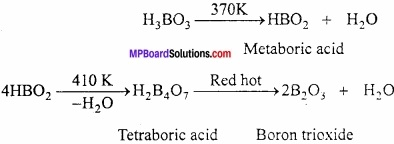Question 2.
Explain the structure of BF3 and BH4, also show the hybridization of B in them?
Answer:
In BF3, there are 3 bonded electron pairs are present in B, so it is of sp2 hybridized and have trigonal planar structure whereas in [BH4] the number of bonding electron = 4. So, the hybridization is sp3 and structure is tetrahedral.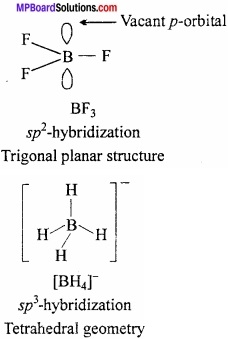Question 3.
What is Alum? Write its general formula, method of preparation, properties and uses?
Answer:
Alums:
Double salts which can be represented by the general formula R2SO4. M2 (SO4)3. 24H2O are called Alums.
Here, R = A monovalent metal like Na, K, Rb, Cs or NH4+ radical.
M = Trivalent metal like Fe, A1 or Cr.
Alums in which trivalent metal is Al, are named as the alums of monovalent metal or radical present in them like:
Potash alum K2SO4. Al2(SO4)3.24H2O
Methods of preparation:
On crystallizing a mixture of equimolecular proportion of potassium sulphate and aluminium sulphate solution.
K2SO4 + Al2(SO4)3 + 24H2O → K2SO4. Al2(SO4)3.24H2O

Properties:
1. Colourless, octahedral crystals are formed whose aqueous solution is acidic due to hydrolysis. Solid alum is soluble in water but insoluble in alcohol. Its one molecule contains 24 molecules of crystallized water.

2. On heating it melts at 92°C, on heating up to 200°C all the water of crystallization is lost and alum swells up and becomes porous. This type of alum is known as burnt alum.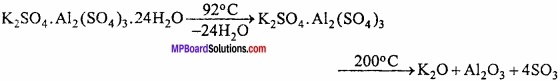Uses:

1. To stop the flow of blood and in medicines. Blood contains negative charge and due to positive charge of Al3+ coagulation takes place which stops bleeding.
2. In dyeing and printing cloth, in sizing of paper.
3. In purifying water.
4. In special foam extinguishers.

Question 4.
Aluminium is a less electrical conductor than copper but aluminium wire is used to make transmission cable. Why?
Answer:
Copper is better conductor, but aluminium is lighter metal, its density is very’ low, so it is better conductor in comparison to copper.

Question 5.
Boron forms only covalent compounds. Why?
Answer:
Due to smaller size and high ionization energy, it has very less tendency to form positive ion. Therefore B cannot release three electrons to form positive ion. (MPBoardSolutions.com) For attaining stable configuration it shares electrons with other atoms and form S covalent bond.Question 6.
Why does boron trihalides behave as a Lewis acid?
Answer:
In BX3 molecule 3 electrons of Boron atom shared with 3 electrons one each from halogen atom. Thus, total number of electrons in the outermost shell of boron trihalides are six which is short of 2 electrons than noble gas electronic configuration. (MPBoardSolutions.com) Thus, BX3 is electron deficient compound and have strong tendency to accept lone pair donated by electron rich compound and form addition compound. Thus, Boron trihalides act as Lewis acid.

Question 7.
Why aluminium cannot be obtained by reduction method from its ore?
Answer:
Aluminium is highly electropositive and behaves as reducing agent. So it can be oxidized easily. On the basis of ionization energy and electron affinity that aluminium behaves as electron donor not electron acceptor. Therefore, it cannot be reduced.

Question 8.
Why is CO gas poisonous?
Answer:
CO combines with haemoglobin of blood and forms a stable compound carbo xyhaemoglobin in which the ability to carry the oxygen of blood is destroyed, due to which the person may become unconscious or even die due to suffocation.

Question 9.
Explain inert pair effect in Boron family?
Answer:
Reason for Inert Pair effect:
As mentioned earlier that tendency to show +1 oxidation state increases down the group. It means the tendency of r – electrons of valence shell to participate in bond formation decreases as we move down the group. (MPBoardSolutions.com) This reluctance of j – electron is termed as inert pair effect. This is due to poor shielding effect of the ns2 electrons by the intervening d – and f – electrons. Another reason for the inert pair effect is that as the size of atom increases from Al to Tl.

The energy required to unpair the ns2 electrons is not compensated by the energy released in forming the two additional bonds. The inert pair effect becomes more predominant as we go down the group because of increased nuclear charge which outweighs the effect of the corresponding increase in atomic size. The electrons thus becomes more tightly hold (more penetrating) and therefore, becomes more reluctant to participate in bond formation.Question 10.
How boric acid is prepared from colemanite ?
Answer:
SO2 gas passed in concentrated aqueous solution of colemanite (Ca2B6O11).
Ca2B6O11 + 2SO2 + 9H2O → 6H3BO3 + 2CaSO3.

Question 11.
What is Borax glass?
Answer:
The anhydrous sodium tetraborate Na2B4O7 is called Borax glass. This is obtained by heating normal boron above its melting point. It is a colourless glass like substance. On absorbing moisture from air converted into decahydrate.
Na2B4O7 + 2H2O ⇄ H2B4O7 + 2NaOH.

Question 12.
What is the effect of heat on borax?
Answer:
Borax when heated strongly loses molecules of water of crystallization and changes into transparent bead.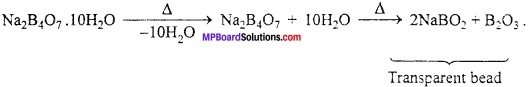Question 13.
How is excessive amount of CO2 responsible for global warming?
Answer:
We know that CO2 is very much essential for plants to carry photosynthesis. The gas is produced during various types of combustion reactions and is released into the atmosphere. It is taken up by plants as pointed above. (MPBoardSolutions.com) Thus, a carbon dioxide cycle works in the atmosphere and its percentage remains nearly constant.

However, over the years, combustion reactions have enormously increased. As a result, CO2 gas is now present in excess in the atmosphere. Like methane, it also behaves like a green house gas and absorbs heat radiated by the earth. Some of the heat is released into the atmosphere while the rest is radiated back to earth. This has resulted in global warming over the years and has brought about major climatic changes.Question 14.
What is the test for Borate radical?
Answer:
Test of Borate radical:
For testing acidic radical borate BO3-3 in laboratory, die given salt is heated with ethanol and concentrated sulphuric acid. Vapours oftriethylborate formed bums with green edged flame. Actually, the salt is first converted into boric acid which then reacts with ethanol forming triethylborate.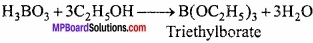Question 15.
Explain the structure of AlCl3?
Answer:
Aluminium trichloride is obtained as a dimer Al2Cl6. There are three electrons in the valence shell of aluminium. These electrons get shared with three electrons of chlorine and form AlCl3. In the valence shell of Al six electrons are present. To complete its octet it requires two electrons. In this condition the Al of AlCl3 takes electrons of Cl of other AlCl3 and octet is completed.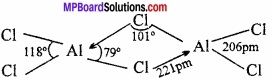Question 16.
What is borax bead test? Explain?
Answer:
In qualitative analysis borax bead test is used for the detection of some coloured ions like Cu2+, Ni2+, CO2+ etc. On heating strongly borax loses water molecule of crystallization and ultimately melts into a transparent bead.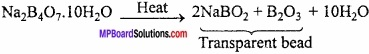B2O3 reacts with certain metal to form metaborates having specific colours. The transparent bead is touched with the speck of the salt. It is then heated in an oxidizing flame and then in reducing flame from the colour of bead in hot and in cold, the basic radical can be predicted.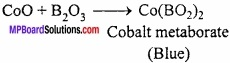Question 17.
Give reason why CCl4 is immiscible in water, whereas SiCl4 is easily hydrolyzed?
Answer:
CCl4 is immiscible in water as it is a covalent compound and is not hydrolysed by water because carbon does not have d – orbitals and hence cannot expand its coordination number beyond 4. However, silicon can expand its coordination number beyond 4 due to availability of d – orbitals.
CCl4 + H2O → No reaction
SiCl4 + 4H2O → Si(OH)4 + 4HCl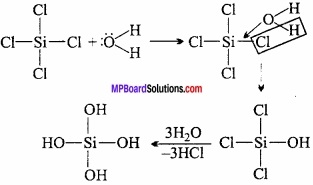Question 18.
Explain why CO2 is a gas whereas SiO2 is a solid. Or, Explain the structure of CO2 and SiO2?
Answer:
The structure of CO2 is linear. The C is sp hybridized and the molecules of CO2 are attached with weak vander Waals forces. That is why at normal temperature CO2 is a gas.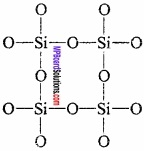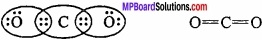SiO2 is solid. Its structure is like lattice crystal. Every Si is joined with O atom tetrahedrally. Covalent single bond is present between Si – O. This bond is stronger than vander Waals forces. That is why SiO2 is solid and have high m.p.

Question 19.
Explain back – bonding with example?
Answer:
There are six electrons in the valence shell of BCl3. Due to electron acceptor BF3 behaves as Lewis acid. It should be a strong Lewis acid but it works as weak Lewis acid.

In BF3 the B is sp2 hybridized, so BF3 is a planar molecule. In this molecule 2p, orbital of B is completely vacant. In the 2pz orbital of fluorine two electrons are present. The 2pz orbitals of B and 2pz orbitals of fluorine overlap and form a bond. This is called back – bonding.

Question 20.
(a) BCl3 is stable but B2Cl6 was not found whereas AlCl3 is unstable. Why?
(b) AlCl3 is unstable, Al2Cl6 is stable? Give the reason?
Answer:
(a) BCl3 is stable as in the valence shell of BCl3 electrons are present but due to back – bonding the resonating structure gives stability to BCl3. In B2Cl6 the d – orbitals of B is not vacant so it cannot accept the electron from chlorine and so it is impossible to form B2Cl6

(b) In valence shell of AlCl3 six electrons are present and due to incomplete octet AlCl3 is unstable. But Al2Cl6 dimer, the vacant d – orbital of Al accept the electrons from chlorine and so octet gets completed. So Al2Cl6 is stable.

Question 21.
Explain the orbital structure of carbon monoxide?
Answer:
Orbital structure of carbon monoxide: Both C and O in CO is in sp hybrid state.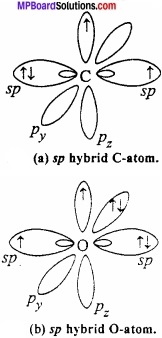One of the sp hybrid orbital of carbon overlaps with sp hybrid orbital of oxygen to form σ bond. One sp – orbital each of carbon and oxygen carries lone pair of electrons which remains non – bonded. Half – filled pz orbital of carbon and oxygen overlaps laterally to form π bond. Now, filled py orbital of oxygen overlaps with vacant py orbital of carbon to form coordinate bond.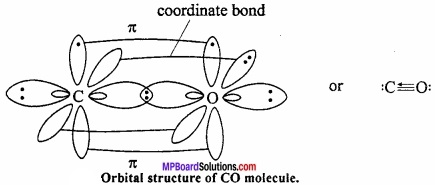Question 22.
What is tetrahedral nature of carbon?
Answer:
The atomic number of C is 6. On the basis of this two electrons are present in first shell and four electrons in second shell. So its valency is 4. (MPBoardSolutions.com) According to Levat and van’t Hoff, if in a centre of tetrahedral it is considered to be C atom then H at four comers covalency of C can be shown. The angle between the two valencies is 109°28′. According to Henry’s experiment the covalencies of C is symmetrical. It is presented as tetrahedral in space not in one plane.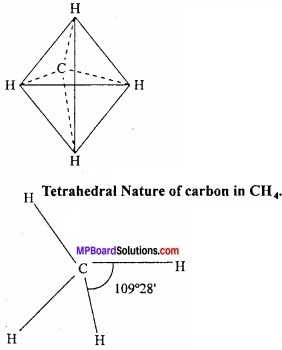Question 23.
Why is graphite used as lubricant?
Answer:
Graphite has layered structure in which the different layers are held together by weak van der Waals forces and hence can be made to slip over one another. Therefore graphite acts as a lubricant.

Question 24.
Why diamond is used as an abrasive?
Answer:
Every carbon in diamond is sp3 hybridized and each carbon form strong covalent bond with other four carbon atoms. In this way the structure of diamond is tetrahedral three dimensional which is very hard. Due to this, diamond is the most hardest element known, therefore it is used as abrasive.

Question 25.
What is silica garden?
Answer:
In sand, crystals of copper sulphate, ferrous sulphate, nickel sulphate, cadmium nitrate, sulphate and cobalt nitrate etc. are added to a saturated solution of sodium silicate in a tube, then after two or three days coloured plants seen to grow in the solution and is known as silica garden.Question 26.
Carbon monoxide exist but silicon monoxide does not. Why?
Answer:
Carbon can form a n bond after forming a a (sigma) bond with oxygen. At the same time vacant 2p, orbital of carbon can overlap with 2p: orbital of oxygen containing a lone pair of electron. (MPBoardSolutions.com) This is possible because carbon possess some electronegativity to gain a lone pair of electron of oxygen. On the other hand, Si atom is bigger in size and its electronegativity is also less due to which it cannot form 3pz – 2pz π bond. That is why SiO is not possible.

Question 27.
If the initial compound in the formation of silicone is RSiCl3, then give the structure of the product?
Answer:
After the hydrolysis of alkyl trichlorosilane and after its polymerisation, chain isomers (silicones) are obtained.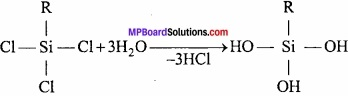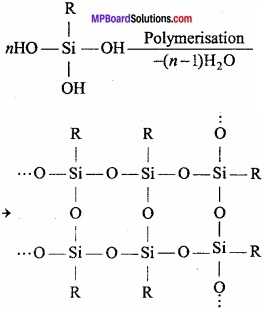Question 28.
What is catenation and in which compound it is found maximum?
Answer:
It is the ability of like atoms to link with one another through covalent bonds. This is found maximum in carbon. This is due to smaller size and higher electronegativity of carbon and unique strength of carbon – carbon bond.

Question 29.
Write the structure of graphite?
Answer:
Structure of graphite:
Carbon atoms in graphite are in sp2 hybrid state. Carbon atoms get bonded to each other forming hexagonal structure. (MPBoardSolutions.com) Layer of hexagonal arrangement of carbon is held together by vander Waals forces. Carbon – carbon bond distance in hexagonal ring is 142 pm while between carbon of two layers it is 340 pm. These layers can slide over each other due to the presence of weak bond.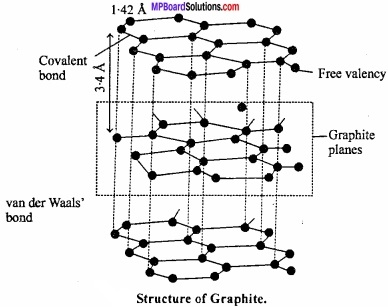Question 30.
Write the balanced equation for preparation of water gas, carburated water gas and producer gas?
Answer:
Water gas:
This is a mixture of CO and H2
C + H2O $$\underrightarrow { 1160^{ \circ }C }$$ [CO + H2] – 29,000 calories.
Carburated water gas:
On passing the water gas into hot bricks present in oil, acetylene and ethylene forms and it mixes with water gas and form carburated water gas. In this, the amount of constituents are: CO = 30%, H2 = 35%, Saturated hydrocarbon = 15.20%, Hydrocarbons = 10%, N2 = 2.5-5%, CO2 = 2%.
Producer gas:
Mixture of CO and N2.
2C + air (O2 + N2) → 2CO + N2

Question 31.
Why carbon cannot form complex compounds whereas other member of group 14 can form? Account for it?
Answer:
The tendency of an element to form complex is favoured by it:

1. High charge density
2. Small size
3. Availability of vacant d – orbital.

From the valence shell electronic configuration of carbon atom it is cleared that it can accompanied maximum of eight electrons in its valence shell while forming covalent bond since carbon atom does not have vacant d – orbital in these tetravalent compound so it cannot form complexes by accomodating any more electron. (MPBoardSolutions.com) On the other hand, in case of other member of group IV are available to their tetracovalent compounds and consequently they can form complexes by accepting lone pair of electron.

p – Block Elements Long Answer Type Questions – I

Question 1.
What is Borane? Write its characteristics and uses?
Answer:
Boron Hydrides or Hydrides of Boron:
Boron forms two series of hydrides. One of its nidoborane with general formula BnHn+4 and other is arachnoborane with general formula BnHn+6. Nidoborane series (BnHn+6): Its first member BH2 does not exist. Second member B2H6 is very important and called as diborane. Other important members are pentaborane (9) B5H6, hexaborane (10) B6H10, octaborane (12) B8H12.

Arachnoborane series (BnHn+6):
Tetraborane B4H10, pentaborane (11) B5H11, hexaborane (12) B6H12 etc. are important members of this series.
Diborane (B2H6):
Diborane is the simplest borane.
Preparation:
(I) By the action of lithium aluminium hydride on boron trichloride in the presence of ether.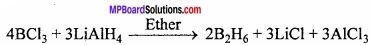(II) By passing the silent electric discharge at low pressure through a mixture of boron trichloride or tribromide and excess of hydrogen.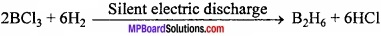(III) Lab preparation:
By the action of iodine on sodium borohydride in diethylene glycol dimethyl ether (diglyme) as solvent.
2NaBH4 + I2 → B2H6 + 2NaI + H2

(IV) Industrial preparation:
Diborane is obtained on large scale by the reduction of BF3 with NaH at 175°C.
2BF3 + 6NaH $$\underrightarrow { 175^{ \circ }C }$$ B2H6 + 6NaF

Physical properties:
It is a colourless gas with foul smell and highly toxic in nature. Uses:

1. As rocket fuel
2. As catalyst in polymerisation reaction.

Question 2.
Explain the structure of diborane? Or, What are electron deficient compounds? Or, What are 2 electron 3 centre compounds?
Answer:
Diborane is prepared by reduction of BF3 with LiH at 450 K.
2BF3 + 6LiH $$\underrightarrow { 400^{ \circ }C }$$ B2H6 + 6LiF
Diborane is also prepared by treatment of BCl3 with LiAlH4.
4BCl3 + 3LiAlH4 → 2B2H6 + 3LiCl + 3AlCl3.

Structure of diborane:
The structure of diborane is determined by electron diffraction studies. According to this structure sixteen electrons are required for the formation of conventional covalent bond whereas in diborane there are only twelve valence electron three from each boron and six from hydrogen. In this way it is an electron deficient compound.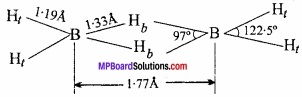Diborane has two coplanar BH2 groups and the remaining two hydrogen atoms lie centrally between BH2 group. In the structure four hydrogen atoms are terminal hydrogen and two hydrogens are bridge hydrogen. The two BH2 groups lie in the same plane while the two bridging hydrogen atoms lie in a plane perpendicular to this plane. (MPBoardSolutions.com) Each bridged hydrogen is bonded to the two atoms only by sharing of two electrons. Such covalent bond is called three centre electron pair bond or multi centre bond.

Question 3.
What happens when:

1. Borax is heated strongly.
2. Boric acid is added to water.

Answer:
1. Borax when heated strongly loses molecules of water of crystallization and changes into a transparent bead.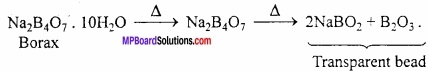2. It is weak monobasic acid. Boric acid is not a proton donor (i.e protic acid). It accepts a pair of electron from water and acts as a Lewis acid (electron acceptor).
B(OH)3 + 2HOH → [B(OH)4] + H3O+

Question 4.
A certain salt X, gives the following results:

1. Its aqueous solution is alkaline to litmus.
2. It swells up to glassy material Y on strong heating.
3. When cone. H2SO4 is added to a hot solution of X, white crystal of an acid Z separates oct?

Write equation for all the above reaction and identify X, Y and Z?
Answer:
1. Since the aqueous solution of salt (X) is alkaline to litmus, it must be the salt of strong base and a weak acid.

2. Since the salt (X) swells up to glassy material Y on strong heating, therefore X must be Borax and Y must be a mixture of sodium metaborate and boric anhydride.

3. When cone. H2SO4 is added to hot solution of X, white crystals of an acid Z separates out. Therefore Z must be orthoboric acid.
Equation for the all above reactions are as follows:

4.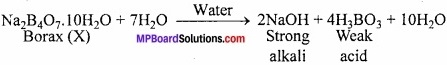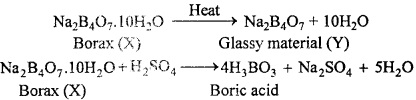Question 5.
Explain the following reaction:

1. Silicon is heated with methyl chloride at high temperature in the presence of copper.
2. Silicon dioxide is treated with hydrogen fluoride.
3. CO is heated with ZnO.
4. Hydrated alumina is treated with aqueous NaOH solution.

Answer:
1. A mixture of m ono, di and trimethylchlorosilances along with a small amount of tetra – methylsilance is formed.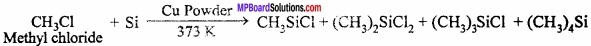2. SiO2 + 4HF → SiF4 + 2H2O
SiF4 + 2HF → H2SiF6

3. ZnO is reduced to zinc metal.
ZnO + CO → Zn + CO2

4. Aluminium dissolves to form sodium meta – aluminate.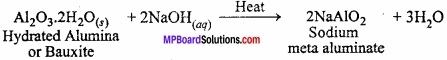Question 6.
Compare Boron and Carbon?
Answer:
Similarities:

1. Boron and carbon are non – metals.
2. Both show allotropy.
3. Both form more than one type of hydride
4. Both form covalent compounds
5. Compounds of both the elements are soluble in organic solvents.

2NaOH + CO2 → Na2CO3 + H2O
2NaOH + B2O3 → Na2B2O3 + H2O

Dissimilarities:

Dissimilarities between Boron and Carbon:
Boron:

1. Electronic configuration is 1s2,2s2,2p1.
2. Valency is 3.
3. Not formed double and triple bond.
4. Electron deficient compounds

Carbon:

1. Electronic configuration is 1s2,2s2,2p1.
2. Valency is 4.
3. It forms double and triple bond.
4. Not electron deficient compounds.Question 7.
Compare the structure of BCl3 and AlCl3?
Answer:
BCl3 is an electron deficient compound which present always as monomer. In BCl3, the B is sp2 hybridized. Therefore, its structure is triagonal and bond angle is 120°. The atomic radius of Boron is small and Chlorine bridge is unstable, therefore, it will not form dimer.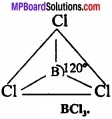AlCl3 is always present as dimer. In this structure, the A1 atom accept lone pair of electron from the Cl atom of another Al atom and octate is completed and attain stability.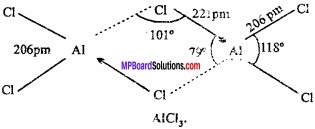Question 8.
Explain the structure of diborane and boric acid?
Answer:
Structure of diaborane:
Diborane LS an example of ‘three centre two electron pair bond’ or banana bond in which two boron atom and one hydrogen atom has only 2 electrons available for bonding. These are two banana bonds of this type in diborane.

Two boron atoms and two hydrogen atoms (Total four hydrogen atom) forming covalent bond with each boron atom lies in the same plane. (MPBoardSolutions.com) Among remaining two hydrogen atom one lies above and other below the plane of boron atoms forming banana bond. These hydrogens are called bridging hydrogen.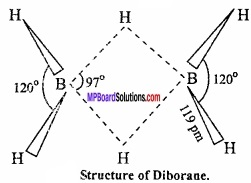Bond length of regular B – H covalent bond is 119 pm.
Bond length of banana B – H bond is 134 pm.
Interatomic distance between two boron atom is 178 pm. The reason for the formation of banana bond is that there are only 12 electrons in diborane (6 from two boron and 6 from six hydrogen) while, for the formation of 8 covalent bonds between 2 boron and 6 hydrogen, total 16 electrons are required. Thus, diborane is an electron deficient compound.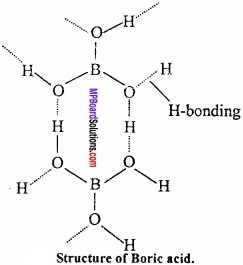Structure:
Crystal of boric acid are soapy in touch and have layer like structure similar to graphite
BO33- is bonded to hydrogen covalentlly and through hydrogen bond.

Question 9.
Describe abnormal behaviour of boron with respect to other elements of group – 13?
Answer:
The first element boron of group-13 differs from the rest elements due to its:

1. Small size
2. High electronegativity
3. Absence of d – orbitals

Abnormal behaviour:

1. Boron is non – metal and others are metals.
2. Boron exhibits allotropy while others do not.
3. Oxides and hydroxides of B are acidic in nature. Oxides of A1 and Ga are amphoteric while articles and hydroxides of In and T1 are basic.
4. Melting point of B is higher in comparison to rest of other elements.
5. Boron combines with metals to form borides. Whereas others do not form borides.
6. B does not form alum, but Al, Ga and In form alums. ,
7. B does not form B+3 but others elements of this group form m+3 ions.
8. B generally forms covalent compounds whereas other elements of the group -13 form both covalent and electrovalent compounds.

Question 10.
Write a not on fullerene?
Answer:
After 1985, new allotropes of carbon were discovered which contains cluster of C32, C30, C60, C70 etc. they are known as fullerene. C60 cluster is of special importance which is known as Buck – minister fullerene.

The structure of fullerene resembles with that of a soccer ball with six membered as well as five membered rings the carbon atom in fullerene have been found to be equivalent and are connected by both single bond and double bond. These are called Bucky Ball. Physical Properties:

1. It is soluble in organic solvent.
2. Its molecule is sufficiently stable.
3. It forms complex with platinum.

Chemical properties:
1. Combustion:
In limited supply of air it forms CO and in complete combustion it forms CO2.
2C + O2 → 2CO + 52 Kcal
C + O2 → CO2 + 94 Kcal

2. Action with metal:
It reacts with metal at high temperature to form carbides.
Ca + 2C → CaC2
4AI + 3C → Al4C3

3. Oxidizing property:
Oxides of many metals are reduced to metal at high temperature.
ZnO + C → Zn + CO
Fe2O3 + 3C → 2Fe + 3CO.

Uses:

1. As Lubricant
2. As conductor.Question 11.
Why carbon shows dissimilarity with the other members of its group?
Answer:
Carbon shows dissimilarity with the other members because the reason is:

1. Atomic radius and ionic radius is small.
2. High ionisation energy.
3. High electron affinity.
4. Absence of d – orbital.

Anomalous behaviour:

1. The melting and boiling points of C is high in comparison to other members.
2. Property of catenation is high.
3. Carbon forms multiple bonds, but other members don’t.
4. Mono – oxide of C is known, but of other members are not found.
5. The maximum valency of C is 4, but other members 6.
6. Carbon does not form compounds like other members.
7. Carbon forms more than one type of hydride.
8. CO2 is a gas, but dioxide of other members are solid.

p – Block Elements Long Answer Type Questions – II

Question 1.
Write short notes on following:

1. Freon
2. Silicone.

Answer:
1. Freon: Dichloro – difluoro methane is known as freon. Freon is formed by the reaction of CCl4 with HF or SbF3 in presence of SbCl5. Freon is used as coolent in refrigerators and A.C.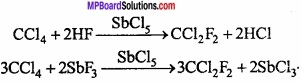2. Silicone:
Silicones are polymers containing R2SiO units. Name silicone is given to it because of the resemblance of formula of its basic unit with that of ketones R2CO. Silicones are also found in form of polymers while ketones can exist as independent molecule as well as polymer.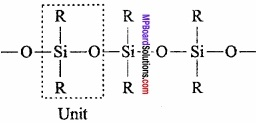Preparation:
Alkyl chloride is heated with silicon in presence of copper powder to produce dialkyl – dichloro – silane.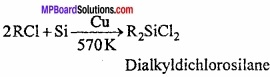This compound on hydrolysis produces dihydroxysilane.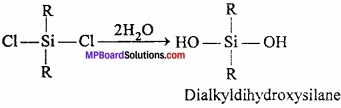Due to the presence of two – OH group on a silicone atom, these molecules condenses together to form polymers.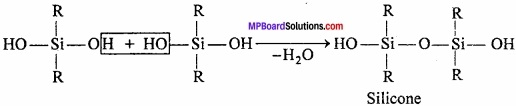In this way, due to condensation of large number of units, unite together. Cross – linking occurs if, trihydroxysilane units are involved and a two – dimensional polymer is formed.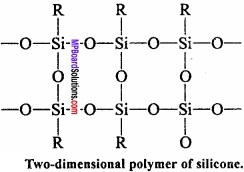Uses:

1. Silicones are insulators, heat resistant, non-reactive and water repellant. Due to this, it is used for making waterproof cloth and paper.
2. As a lubricant.
3. As insulators in electric appliances.
4. Silicone oil is used in high temperature thermostate and vacuum pumps.

Question 2.
Explain Cold – schmidt alumino thermic process with a labelled diagram? Or, Explain the thermite welding process?
Answer:
Thermite process:
It is also called Gold – schmidt aluminothermic process. In this process, refractory crucible is filled with oxide of the metal to be reduced, aluminium powder and barium peroxide. After filling magnesium ribbon it is fixed in sand. (MPBoardSolutions.com) Now, the magnesium ribbon is set on fire. A very high temperature is produced at which metal oxide get reduced by aluminium and collected in molten state. Reaction is extremely exothermic and the 02 required is supplied by barium peroxide. Selection of reducing metal is done on the basis of nature of ore.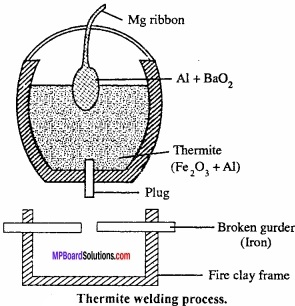Cr2O3 + 2Al → Al2O3 + 2Cr + Heat
Fe2O3 + 2Al → 2Fe + Al2O3

Question 3.
Write short notes on zeolites?
Answer:
These are micro porous aluminosilicate having general formula Mx/nn+[AlO2]x[SiO2]yx- mH2O. Aluminosilicates are obtained by substituting some of the silicon atoms in the three dimensional network of silicon – dioxide by Al atoms. The negative charge carried by aluminosilicate, framework is neutralized by exchangeable cations such as Na+, K+ or Ca2+ of valence n, while m water molecule (mH2O) fill the voids.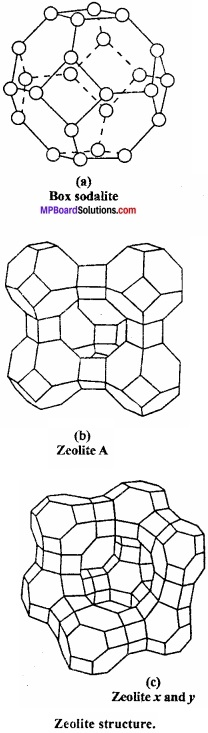Uses:

1. For the manufacture of cement and bricks.
2. In porcelain and glass industries.
3. In pottery industries.

Question 4.
Write similarities and dissimilarities between Boron and Aluminium?
Answer:
Similarities:
1. Both possess similar outer electronic configuration ns2np1.

2. B and Al both are trivalent due to presence of 3 electron in outermost shell.

3. Both form trioxide with oxygen.
4B + 3O2 → 2B2O3
4Al + 3O2 → 2Al2O3.

4. Boron and aluminium both react with base and form H2 gas.
2B + 6NaOH → 2Na3BO3 + 3H2
2Al + 2NaOH + 2H2O → 2NaAlO2 + 3H2.

5. Boron and aluminium both form similar type of compounds with alkyl radicals.
2BCl3 + 3Zn (CH3)2 → 2B(CH3)3 + 3ZnCl2
2Al + 3Hg(CH3)2 → 2Al(CH3)3 + 3H2.

6. Nitride of both are hydrolyzed and give ammonia.
BN + 3H2O → H3BO3 + NH3
AIN + 3H2O → Al(OH)3 + NH3.

Dissimilarities:

Boron:

1. It is a non – metal with three dimensional structure.
2. It exhibits allotropy.
3. It does not form tripositive B+3 ion.
4. B2O3 is acidic in nature.
5. It forms stable hydride.
6. It reacts with metals and form borides.
7. It is bad conductor of electricity.
8. Maximum covalency of boron is 4.

Aluminium:

1. Aluminium is a metal with metallic structure.
2. It does not exhibit allotropy.
3. It forms tripositive Al+3 ion.
4. Al2O3 is amphoteric.
5. It forms unstable hydride.
6. It forms alloys.
7. It is good conductor of electricity.
8. Maximum co valency of Aluminium is 6.Question 5.
Write short notes on:

1. Sodium zeolite
2. Sodium silicate.

Answer:

1. Sodium zeolite:
Sodium zeolite is also known as sodium permutit. Permutit is mixed silicate of sodium and aluminium. Its formula is:
Na2[Al3Si2O8.xH2O]
Sodium zeolite is used in converting hard water into soft water. Water containing calcium and magnesium ion is known as hard water. (MPBoardSolutions.com) When hard water is passed through permutit kept in a column then calcium and magnesium ions are displaced by sodium. Sodium salts do not make water hard and this way soft water is obtained. If sodium permutit is represented by Na2P then the following reaction can be written for softening of water.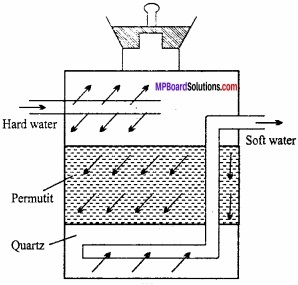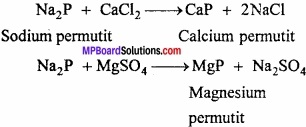2. Sodium silicate or water glass:
It is bright like glass and soluble in water, therefore, it is known as water glass. It contains sodium meta – silicate and silica in excess and its formula is Na2SiO3. SiO2 or Na2SiO3.3SiO2.
It is obtained by fusing a mixture of sodium carbonate and sand.
Na2CO3 + 2SiO2 → Na2SiO3.SiO2 + CO2
The product obtained is hard, solid like glass and is soluble in water.
If sand, crystals of copper sulphate, ferrous sulphate, nickel sulphate, cadmium nitrate, manganese sulphate and cobalt nitrate etc. are added to a saturated solution of sodium silicate in a tube, then after two or three days coloured plants seem to grow in the solution and this is known as silica garden.

Question 6.
Describe, diagonal relationship between B and Si?
Answer:
B and Si show resemblances in properties. The resemblances arise due to the fact that both B and Si are non-metals and have small difference in size. Their following properties are similar:

1. Both are non – metal, non – conductors unable to form cations and possess high melting point.
2. B and Si both do not exist in free state in nature and only exist in the form of their oxides.
3. Both do not react with dilute acid.
4. Both react with nitrogen at high temperature to form nitrides.
2B + N2 → 2BN
Si + 2N2 → SiN4
5. Both form covalent hybride.
6. Both react with metals to form boride and silicates.
7. Both form covalent chlorides which are hydrolyzed in aqueous solution.
BCl3 + 3H2O → H3BO3 + 3HCl
SiCl4 + 3H2O → H2SiO3 + 4HCl
8. H3BO3 and H4Si4 are very weak acids.

Question 7.
What is Alum? Write its general formula, method of preparation and properties?
Answer:
Double salts which can be represented by the general formula R2SO4.M2(SO4)3. 24H2O are called alums.
Here, R = Monovalent metal, like: Na, K, NH4+
M = Trivalent metal, like Fe, Cr, Al Nomenclature of alum:

1. The alum which contains trivalent ion aluminium is called by name of monovalent ion.
K2SO4.Al2(SO4)3.24H2O (Potash alum)

2. The alum which does not contain aluminium as trivalent ion is called by name of both ion.
K2SO4.Fe2(SO4)3.24H2O (Potash ferric alum)

Preparation method:
The crystal of alum are obtained when hot solution of equimolecular quantities of K2SO4 and Al2(SO4)3 are mixed and resulted solution is cooled.
K2SO4 + Al2(SO4)3 + 24H2O → K2SO4.Al2(SO4)3.24H2O

Properties:
Alums are octahedral crystalline compounds. Potash alum is milky in colour. It forms acidic solution in water. It is soluble in water but insoluble in organic solvent.

Effects of heat:
On heating at 365 K it looses water molecule. Its water of crystallization is lost and alum swells up and becomes porous. This type of alum is known as burnt alum.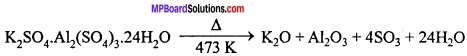Uses:

1. As blood coagulates.
2. In purifying water.
3. In dyeing and printing clothes.
4. In sizing of paper.Question 8.
Write the diagonal relationship between Be and Al?
Answer:
Diagonal Relationship of Beryllium with Aluminium; Beryllium shows diagonal relationship with aluminium which is present in third period and third group (13) of periodic table.
Diagonal relationship between beryllium and aluminium is due to the following reason: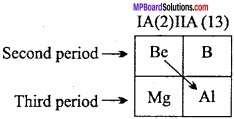1. Nearly equal atomic and ionic radius.
2. Similar electronegativity.
3. Nearly equal electropositivity.
4. Nearly equal polarization power.

Similarities between Be and Al are as follows:
1. Be and Al forms covalent compounds which are soluble in organic solvents.

2. Be and Al oxides are solids having high melting point. They react both with acid and base to produce salt and water due to their amphoteric nature.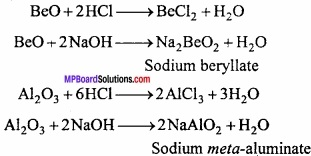3. Be and Al reacts with carbon on heating to form respective carbides which are covalent in nature. Be2C and Al4C3.

4. Carbides of Be and Al forms methane on hydrolysis.
Be2C + 4H2O → CH4 + 2Be(OH)2
Al4C3 + 12H2O → 3CH4 + 4Al(OH)3

5. Be and A1 forms complex fluoro ion in solution.
[BeF4]2- and [AlF6]3-

6. Polymeric structure are found in BeCl2 and AlCl3

7. Solubility of halide of Be and Al is same.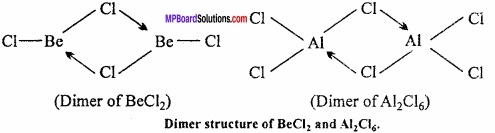8. Be and A1 develops a protective oxide layer on the surface when treated with nitric acid. Due to this, they are not easily attacked by acids.

9. Hydroxide of Be and Al are amphoteric in nature. They react with acid and base to produce salt and water.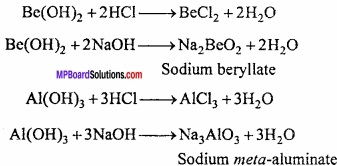Question 9.
What do you mean by:

1. Inert pair effect.
2. Allotropes.
3. Catenation.

Answer:
1. Inert pair effect:
When the tendency of 5 – electrons to be with 5 – electrons and they do not take part in reactions then this tendency is called inert pair effect. The reason is that the tendency to show +1 oxidation state increases down the group. (MPBoardSolutions.com) It means the tendency of 5 – electron of valence shell to participate in bond formation. Thus, among the heavier elements of p – block, the electron pair in the outer 5 – orbitals is reluctant to take part in chemical bonding. It is called inert pair effect.

2. Allotropes:
If an element exists in different physical forms having different properties than the various forms are called allotropes and the phenomenon is called allotropy.
Example:
Crystalline allotropes of C are diamond, graphite and fullerene.

3. Catenation:
It is the ability of like atoms to link with one another through cova¬lent bonds. This is due to smaller size and higher electronegativity of carbon atom and unique strength of C – C bond.
C >> Si > Ge > Sn >> Pb